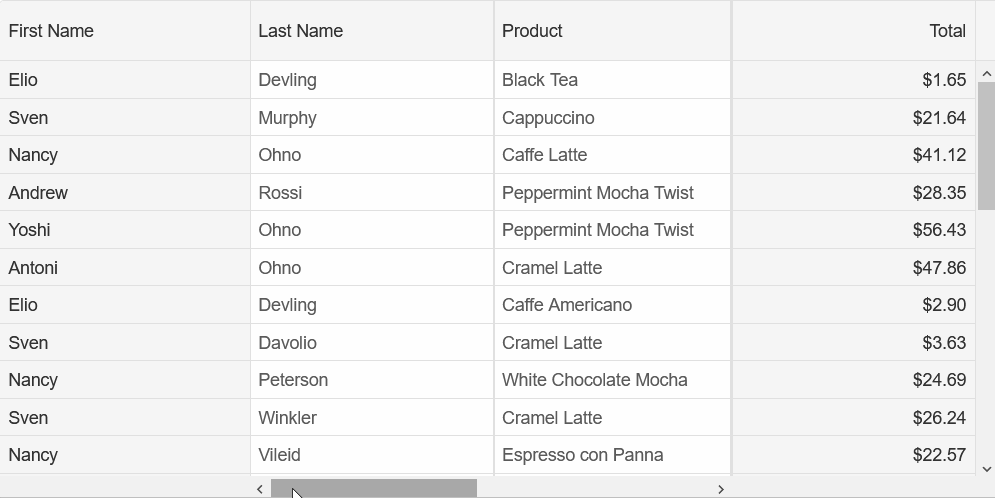Blazor Grid - Frozen Column

## Frozen Column

Smart.Grid allows you to freeze columns, meaning that they will always be visible to the user. Frozen columns can be pinned either `near`(left) or `far`(right).
In the following example, FirstName is pinned to the left and Total is pinned to the right:

```<Grid DataSource="@dataSource" >
<Columns>
<Column DataField="FirstName" Label="First Name" Width="200" Freeze="near"> </Column>
<Column DataField="LastName" Label="Last Name"> </Column>
<Column DataField="ProductName" Label="Product"> </Column>
<Column DataField="Quantity" Label="Quantity" DataType="number"> </Column>
<Column DataField="Price" Label="Unit Price" DataType="number"> </Column>
<Column DataField="Total" Label="Total" DataType="number" Freeze="far"> </Column>
</Columns>
</Grid>```

Example of Grid without Frozen Columns:Example of Grid with Frozen Columns to the left and right: